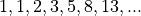# Fibonacci Player Class¶

This section will describe a player who has an internal state that can be modeled using methods and simple values instead of state objects.

This is a variation on the Martingale System. See Martingale Player Design for more information.

In Fibonacci Player Analysis we’ll look at what this player does.

We’ll turn to how the player works in PlayerFibonacci Design.

In Fibonacci Player Deliverables we’ll enumerate the deliverables for this player.

## Fibonacci Player Analysis¶

A player could use the Fibonacci Sequence to structure a series of bets in a kind of cancellation system. The Fibonacci Sequence isAt each loss, the sum of the previous two bets is used, which is the next number in the sequence. In the event of a win, we revert to the basic bet.

Example. Here’s an example of the Fibonacci system.

1. Bet 1. A win.

2. Bet 1. A loss. The next value in the sequence is 1.

3. Bet 1. A loss. The next value in the sequence is 2.

4. Bet 2. A loss. The next value in the sequence will be 3

5. Bet 3. In the event of a loss, the next bet is 5. Otherwise, the bet is 1.

State. In order to compute the Fibonacci sequence, we need to retain the two previous bets as the player’s state. In the event of a win, we revert to the basic bet value of `1`.

In the event of a loss, we can update the two numbers to show the next step in the sequence. The player’s state is defined by these two numeric values.

## PlayerFibonacci Design¶

class `PlayerFibonacci`

`PlayerFibonacci` uses the Fibonacci betting system. This player allocates their available budget into a sequence of bets that have an accelerating potential gain.

### Fields¶

`PlayerFibonacci.``recent`

This is the most recent bet amount. Initially, this is 1.

`PlayerFibonacci.``previous`

This is the bet amount previous to the most recent bet amount. Initially, this is zero.

### Constructors¶

`PlayerFibonacci.``__init__`(self, table: Table) → None

Initialize the Fibonacci player.

Parameters

table (`Table`) – The `Table` object which will accept the bets.

### Methods¶

`PlayerFibonacci.``win`(self, bet: Bet) → None
Parameters

bet (`Bet`) – The bet which won

Uses the superclass method to update the stake with an amount won. It resets `recent` and `previous` to their initial values of 1 and 0.

`PlayerFibonacci.``lose`(self, bet: Bet) → None

Uses the superclass method to update the stake with an amount lost. This will go “forwards” in the sequence. It updates `recent` and `previous` as follows.Parameters

bet (`Bet`) – The `Bet` which lost.

## Fibonacci Player Deliverables¶

There are three deliverables for this exercise.

## Looking Forward¶

We’ve looked at a number of individual class design and construction techniques. At this point, we have a comprehensive simulation of the game of Roulette with a variety of betting strategies. We can run simulations of the various techniques and learn the house always wins. The open question is how large is the house’s edge.

In the next chapter, we’ll wrap up this game by observing some OO design principles and design patterns. This will set the stage for tackling the next game, Craps.